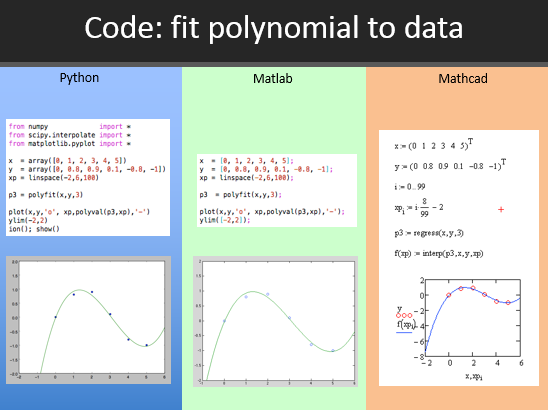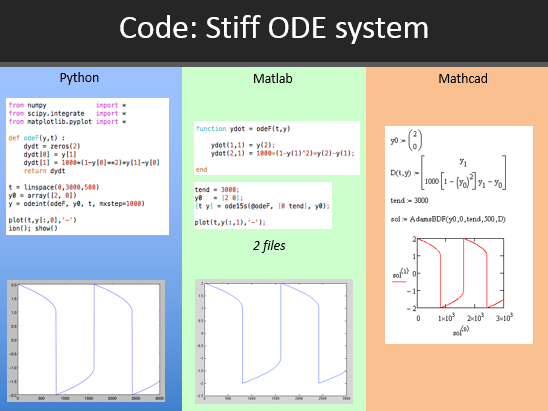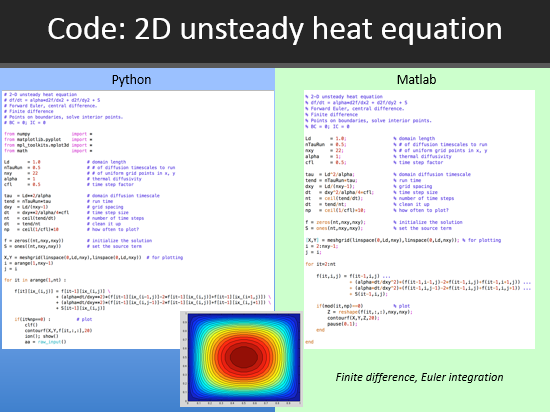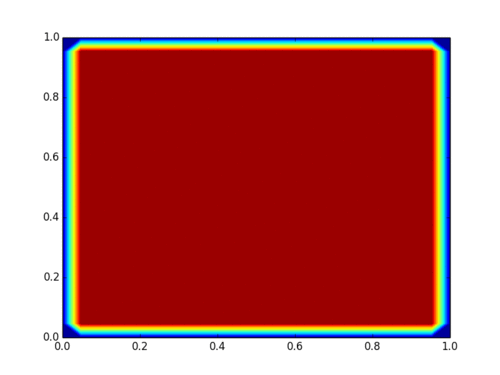## Compare Computational Tools: Python, Matlab, Mathcad

This side-by-side comparison of Python, Matlab, and Mathcad allows potential users to see the similarities and differences between these three computational tools. Each of these tools is reviewed in additional detail through-out the course. For the course projects, any language can be selected. The purpose of these illustrative examples is to demonstrate that these three tools have similar basic capabilities and give insight into which computational tool to select for a project. The following basic methods are demonstrated with sample code in Python, Matlab, and Mathcad.Download Source Code
• Interpolation
• Polynomial fit
• Stiff ODE system
• Integrate function
• Nonlinear equations
• 2D heat equation: Matlab/Python only
• View Full Comparison (PDF)